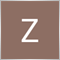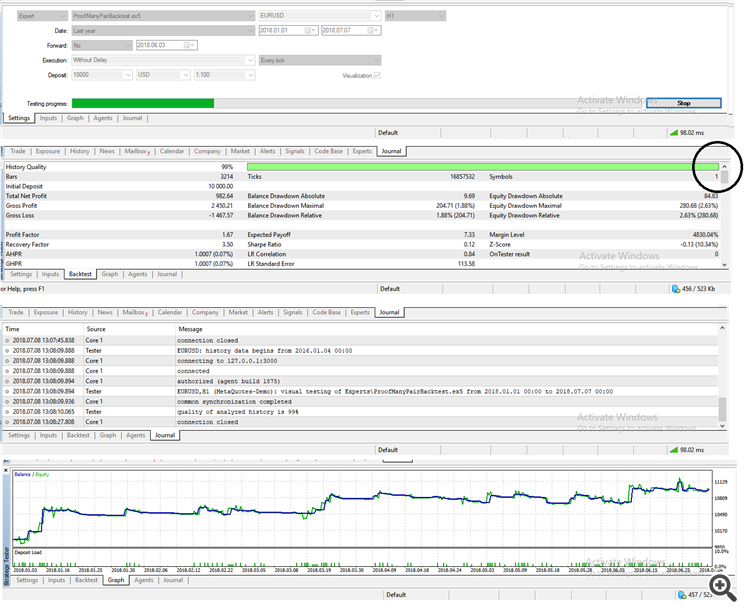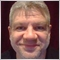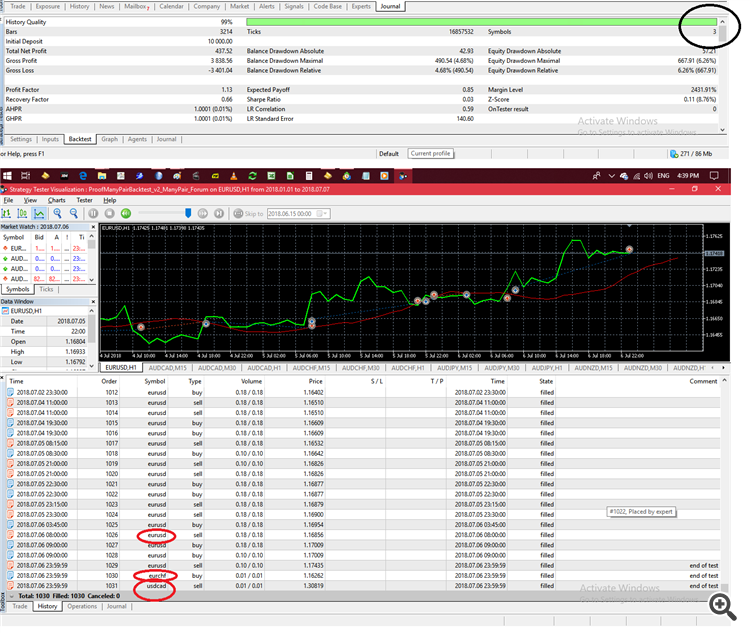# About Testing MultiCurrencies and Time Frames in Strategy Tester174

I have read in the documentation that it is possible to test many currencies (and timeframes?) in MQL5 strategy tester, but could not find a clear and simple example...

So I made minimum changes to an EA that comes along with MT5: Moving Averages.mq5, to include a scanning algorithms...

Well it does goes to testing, but shows just one Symbol (as highlighted in the following picutre):"My" code is:

```//+------------------------------------------------------------------+
//|                                              Moving Averages.mq5 |
//|                   Copyright 2009-2017, MetaQuotes Software Corp. |
//|                                              http://www.mql5.com |
//+------------------------------------------------------------------+
#property version   "1.00"

input double MaximumRisk      = 0.02;   // Maximum Risk in percentage
input double DecreaseFactor   = 3;      // Descrease factor
input int    MovingPeriod     = 12;     // Moving Average period
input int    MovingShift      = 6;      // Moving Average shift
//---
int    ExtHandle=0;
bool   IsHedge=true;   // No worry: Will be determined automatically!

string          ThisP  = _Symbol;
ENUM_TIMEFRAMES ThisT  = _Period;

//+------------------------------------------------- [Multi Filling]-+
string AllPair[] = {
"EURAUD", "EURCAD", "EURCHF", "EURGBP", "EURJPY", "EURNZD", "EURUSD",
"GBPAUD", "GBPCAD", "GBPCHF", "GBPJPY", "GBPNZD", "GBPUSD", "NZDJPY",
ENUM_TIMEFRAMES AllTime[] = {PERIOD_M15, PERIOD_M30, PERIOD_H1};
int p = ArraySize(AllPair);
int t = ArraySize(AllTime);

#define MA_MAGIC 1234501
//+------------------------------------------------------------------+
//| Calculate optimal lot size                                       |
//+------------------------------------------------------------------+
{
double price=0.0;
double margin=0.0;
//--- select lot size
return(0.0);
if(margin<=0.0)                                           return(0.0);
double lot = NormalizeDouble(AccountInfoDouble(ACCOUNT_MARGIN_FREE)  *
MaximumRisk/margin,2);  // Simply free margin x 0.01 risk
if(DecreaseFactor>0) //---    calc number of consecutive losing orders
{
HistorySelect(0,TimeCurrent());    //--- select history for access
int    orders=HistoryDealsTotal(); // total history deals
int    losses=0;                   // nb of consecutive losing orders
for(int i=orders-1;i>=0;i--)       // looping the total history deals
{
ulong ticket=HistoryDealGetTicket(i);
if(ticket==0)
{
break;
}
if(HistoryDealGetString(ticket,DEAL_SYMBOL)!=P)//check symbol
continue;                     // Filter to Keep Our Magic number :
if(HistoryDealGetInteger(ticket,DEAL_MAGIC)!=MA_MAGIC)
continue;                    // Increase consecutive losing   nb:
double profit=HistoryDealGetDouble(ticket,DEAL_PROFIT);
if(profit>0.0) break;
if(profit<0.0) losses++;
} // So teh lot size is 'punished' by taking out third of losses...
if(losses>1)  lot = NormalizeDouble(lot-lot*losses/DecreaseFactor,1);
}
//--- normalize and check limits
double stepvol = SymbolInfoDouble(P,SYMBOL_VOLUME_STEP);
lot = stepvol*NormalizeDouble(lot/stepvol,0); //rounding...
double minvol  = SymbolInfoDouble(P,SYMBOL_VOLUME_MIN);
if(lot<minvol) lot=minvol;
double maxvol  = SymbolInfoDouble(P,SYMBOL_VOLUME_MAX);
if(lot>maxvol) lot=maxvol;
}
//+------------------------------------------------------------------+
//| Check for open position conditions                               |
//+------------------------------------------------------------------+
void CheckForOpen(string P, ENUM_TIMEFRAMES T)
{
MqlRates rt;       //--- go trading only for first ticks of new bar
if(CopyRates(P,T,0,2,rt)!=2)
{Print("CopyRates of ",P," failed, no history");              return;}
if(rt.tick_volume>1)                                       return ;
double   ma;                       //--- get current Moving Average
if(CopyBuffer(ExtHandle,0,0,1,ma)!=1)
{ Print("CopyBuffer from iMA failed, no data");              return;}
//--- check signals
ENUM_ORDER_TYPE signal=WRONG_VALUE;
if(rt.open>ma &&rt.close<ma) signal=ORDER_TYPE_SELL;//sell
else
}
if(signal!=WRONG_VALUE)
Bars(P,T)>100)
SymbolInfoDouble(P,
}
//+------------------------------------------------------------------+
//| Check for close position conditions                              |
//+------------------------------------------------------------------+
void CheckForClose(string P, ENUM_TIMEFRAMES T)
{
MqlRates rt;       //--- go trading only for first ticks of new bar
if(CopyRates(P,T,0,2,rt)!=2)
{Print("CopyRates of ",P," failed, no history");              return;}
if(rt.tick_volume>1)                                        return;
double   ma;                       //--- get current Moving Average
if(CopyBuffer(ExtHandle,0,0,1,ma)!=1)
{Print("CopyBuffer from iMA failed, no data");                return;}
bool signal=false; //--- positions already selected before
long type=PositionGetInteger(POSITION_TYPE);
&& rt.close<ma)    signal=true;
if(type==(long)POSITION_TYPE_SELL && rt.open<ma
&& rt.close>ma)   signal=true;
{
}
}
//+------------------------------------------------------------------+
//| Position select depending on netting or hedging                  |
//+------------------------------------------------------------------+
bool SelectPosition(string P)
{
if(IsHedge)                      //--- check position in Hedging mode
{
uint total=PositionsTotal();
for(uint i=0; i<total; i++)
{
string P_Poç = PositionGetSymbol(i);
if(P==P_Poç && MA_MAGIC==PositionGetInteger(POSITION_MAGIC))
{
answer=true;         // So you may continue selecting the ticket...
break;
}
}
}
else                              //--- check position in Netting mode
{
if(!PositionSelect(P))
return(false);
else
return(PositionGetInteger(POSITION_MAGIC)==MA_MAGIC);
}

return(answer); //--- result for Hedging mode (above not returned yet)
}
//+------------------------------------------------------------------+
//| Expert initialization function                                   |
//+------------------------------------------------------------------+
int OnInit(void)
{
//Prepare trade class to control positions if hedging mode is active
// first determine automatically if we are in hedging account        :
IsHedge=((ENUM_ACCOUNT_MARGIN_MODE)AccountInfoInteger(
ACCOUNT_MARGIN_MODE)==ACCOUNT_MARGIN_MODE_RETAIL_HEDGING)  ;

//--- Moving Average indicator
ExtHandle=iMA(ThisP,ThisT,MovingPeriod,MovingShift,MODE_SMA,
PRICE_CLOSE);
if(ExtHandle==INVALID_HANDLE)
{
printf("Error creating MA indicator");
return(INIT_FAILED);
}
return(INIT_SUCCEEDED);
}
//+------------------------------------------------------------------+
//| Expert tick function                                             |
//+------------------------------------------------------------------+
void OnTick(void)
{
for(int i=0; i<p; i++)
for(int j=0; j<t; j++)
{
ThisP  = AllPair[j]                                                ;
ThisT  = AllTime[t]                                                ;
if(SelectPosition(ThisP))
CheckForClose(ThisP,ThisT)                                         ;
else
CheckForOpen(ThisP,ThisT)                                          ;
}
}
//+------------------------------------------------------------------+
//| Expert deinitialization function                                 |
//+------------------------------------------------------------------+
void OnDeinit(const int reason)
{
}
//+------------------------------------------------------------------+
```42179

I have read in the documentation that it is possible to test many currencies (and timeframes?) in MQL5 strategy tester, but could not find a clear and simple example...

So I made minimum changes to an EA that comes along with MT5: Moving Averages.mq5, to include a scanning algorithms...

Well it does goes to testing, but shows just one Symbol (as highlighted in the following picutre):

"My" code is:

Don't know how you get a result with this code, it contains a critical error :

```for(int i=0; i<p; i++)
for(int j=0; j<t; j++)
{
ThisP  = AllPair[j]                                                ;
ThisT  = AllTime[t]                                                ;
```

Your MA handle is initialized on chart symbol only.

Beside that, your way to check for a new bar is incorrect.174

Thank you Mr. Varleyen

Yes it was a bad typo as I was copy pasting from code to code...

I corrected the loop parameters (not in hurry now to solve the New Bar issue) and definitely saw improvements:As seen in this picture, the Back test analyzed  all the currencies, however traded only in three of them, with a big preference for the first chart pair (EURUSD)

Is this normal? or is it something related to my code?